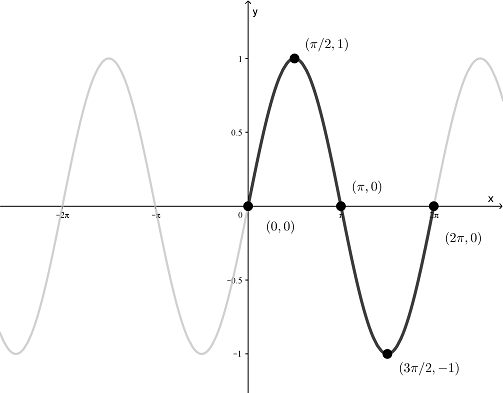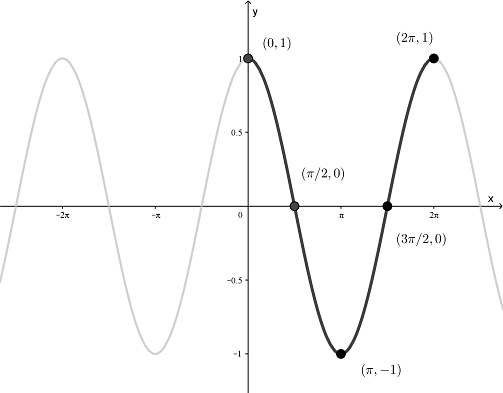# Section 1.5 Graphs of Sine and Cosine Functions

Section Objectives

1. Sketch the graphs of general sine and cosine functions.

### Basic Graphs

Before we even start sketching the graphs of $y=\sin x$ and $y=\cos x$, we should recall a few important ideas about these functions.

• Domain: Each is defined for all real numbers, $-\infty < x < \infty$.
• Range: Each has output values between -1 and 1, $-1 \le y \le 1$.
• Periodic: Each is periodic with period $2\pi$. The graph repeats every $2\pi$ units.
• Symmetry: Each function exhibits a kind of symmetry that can be seen from the unit circle approach. In fact, $\sin(-x) = -\sin(x)$ (odd symmetry) and $\cos(-x) = \cos(x)$ (even symmetry).
• Points: A number of specific points on the graph of each function can be obtained from the unit circle.

The graph of $y=\sin x$ is shown here.The graph of $y=\cos x$ is shown here.### More General Graphs

Look carefully for the key ideas that were outlined above. By thinking about how those ideas change under transformations, we can easily graph the general sine and cosine functions:

$y = d + a \sin(bx-c)$

$y=d+a\cos(bx-c)$

#### Examples

• Sketch the graph of $y=2 \sin x$

• Sketch the graph of $y=-\cos x$

• Sketch the graph of $y=1-\cos x$

### Period, Amplitude, and Phase Shifts

The graphs of $y=d+a \sin(bx-c)$ and $y=d+a \cos(bx-c)$ have the following characteristics (assuming $b>0$):

• Amplitude $=|a|$. The vertical distance from low point to high point is $2|a|$.
• Period $=2\pi/b$.
• Phase shift $=c/b$. (Positive for right. Negative for left.)
• The left and right endpoints of one period can be determined by solving $bx-c=0$ and $bx-c=2\pi$.
• The highest point on the graph (max. value) has $y$-coordinate $y=d+|a|$, and the lowest point on the graph (min. value) has $y$-coordinate $y=d-|a|$.

As a general rule of thumb, we can sketch the graphs of more general sine and cosine functions by using this procedure:

1. Sketch the graph of the corresponding basic function without the axes scaled.
2. Scale the axes to satisfy the characteristics described above.
3. Redraw, if necessary, to make a "cleaner" graph.

It is sometimes useful to write

$\displaystyle y=d+a \sin(bx-c) = d + a \sin\left[ b \left( x - \frac{c}{b} \right) \right]$

or

$\displaystyle y=d+a \cos(bx-c) = d + a \cos\left[ b \left( x - \frac{c}{b} \right) \right]$

#### Examples

• Sketch the graph of $\displaystyle y=\frac{1}{2} \sin \left( x - \frac{\pi}{3} \right)$.

• Sketch the graph of $\displaystyle y=-3+2 \cos\left(3x-\frac{\pi}{2} \right)$.

• Write an equation whose graph has the following characteristics: a cosine curve with period $\pi$, amplitude 3, left phase shift $\pi/4$, and vertical translation down 1 unit.